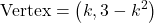Question

If k is a non-zero constant, determine the vertex of the function y = x2 – 2kx + 3 in terms of k.

1.Calantha

Set y = 0, evaluate the quadratic at h=−b2a and solve for k.

k=9 I think….. I’m not completely sure but I think that’s how it is

2.thanhha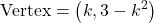Step-by-step explanation:

We are given the quadratic equation: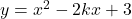Where k is a non-zero constant.

And we want to determine the vertex of the parabola in terms of k.

The vertex of a parabola is given by the formulas: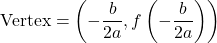In this case, a = 1, b = -2k, and c = 3.

Find the x-coorinate of the vertex: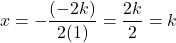To find the y-coordinate, we substitute the value we acquired back into the equation. So: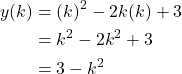Therefore, our vertex in terms of k is: